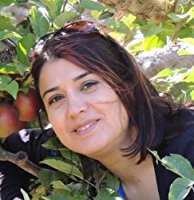﻿ Atife CaglarI moved  to Houston on August 2016 because of a family relocation. Previously I was a tenured Associate Proffessor at University of Wisconsin-Green Bay where I taught over ten years.

Courses taught at University of Houston

MATH 1313 Finite Mathematics with Applications
MATH 1330 Precalculus
MATH 1432 Calculus 2
MATH 1450 Honors Calculus ( Calculus 1&2)
MATH 1451 Honors Calculus ( Multivariate Calculus)
MATH 3321 Engineering Mathematics (online and face to face)
MATH 3325 Transition to Advanced Mathematics
MATH 3330 Abstract Algebra
MATH 3331 Differential Equations
MATH 3363 Introduction to Partial Differential Eqautions
MATH 3399 Senior Honors Thesis

Teaching  Spring 2020

MATH 1313 Finite Mathematics  (all course material are in CASA)

MATH 3363 Partial Differential Equations ( all course material are in Blackboard)

MATH 3321 Enginering Mathematics (all course material are in CASA)

MATH 3321

In order to see the list of topics (sections) that will be covered in each test please read this link

Chapter 1                        Chapter 1   Completed

# Chapter 2              Chapter 2 Completed

Chapter 3 Part 2           Chapter 3 Part 2 Completed

EMCFs Quizz Procedure   EMCFs  are posted on CASA

Review Questions for TEST 1